Custom SearchLIKE AND UNLIKE FRACTIONS We have shown that like fractions are added by simply adding the numerators and keeping the denominator. Thus,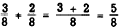orSimilarly we can subtract like fractions by subtracting the numerators.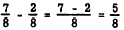The following examples will show that like fractions may be divided by dividing the numerator of the dividend by the numerator of the divisor.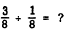SOLUTION: We may state the problem as a question: How many times does 1/8 appear in 3/8 or how many times may 1/8 be taken from 3/8?"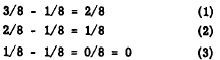We see that l/8 can be subtracted from 3/8 three times. Therefore, 3/8 1/8 = 3 When the denominators of fractions are unequal, the fractions are said to be unlike. Addition, subtraction, or division cannot be performed directly on unlike fractions. The proper application of the fundamental rule, however, can change their form so that they become like fractions; then all the rules for like fractions apply. LOWEST COMMON DENOMINATOR To change unlike fractions to like fractions, it is necessary to find a COMMON DENOMINATOR and it is usually advantageous to find the LOWEST COMMON DENOMINATOR (L C D). This is nothing more than the least common multiple of the denominators. Least Common Multiple If a number is a multiple of two or more different numbers, it is called a COMMON MULTIPLE. Thus, 24 is a common multiple of 6 and 2. There are many common multiples of these numbers. The numbers 36, 48, and 54, to name a few, are also common multiples of 6 and 2. The smallest of the common multiples of a set of numbers is called the LEAST COMMON MULTIPLE. It is abbreviated LCM. The least common multiple of 6 and 2 is 6. To find the least common multiple of a set of numbers, first separate each of the numbers into prime factors. Suppose that we wish to find the LCM of 14, 24, and 30. Separating these numbers into prime factors we have 14 =2 x 7 24 = 23 x 3 30 = 2 x 3 x 5 The LCM will contain each of the various prime factors shown. Each prime factor is used the greatest number of times that it occurs in any one of the numbers. Notice that 3, 5, and 7 each occur only once in any one number. On the other hand, 2 occurs three times in one number. We get the following result: LCM = 23 x 3 x 5 x 7           = 840 Thus, 840 is the least common multiple of 14, 24, and 30. Greatest Common Divisor The largest number that can be divided into each of two or more given numbers without a remainder is called the GREATEST COMMON DIVISOR of the given numbers. It is abbreviated GCD. It is also sometimes called the HIGHEST COMMON FACTOR. In finding the GCD of a set of numbers, separate the numbers into prime factors just as for LCM. The GCD is the product of only those factors that appear in all of the numbers. Notice in the example of the previous section that 2 is the greatest common divisor of 14, 24, and 30. Find the GCD of 650,900, and 700. The procedure is as follows: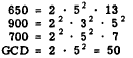Notice that 2 and 52 are factors of each number. The greatest common divisor is 2 x 25 = 50. USING THE LCD Consider the example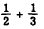The numbers 2 and 3 are both prime; so the LCD is 6. Therefore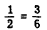and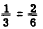Thus, the addition of \$ and i is performed as follows:In the example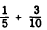10 is the LCD. Therefore,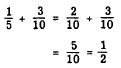Practice problems. Change the fractions in each of the following groups to like fractions with least common denominators: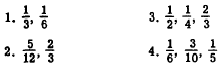Answers: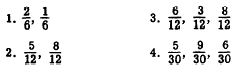Integrated Publishing, Inc. - A (SDVOSB) Service Disabled Veteran Owned Small Business